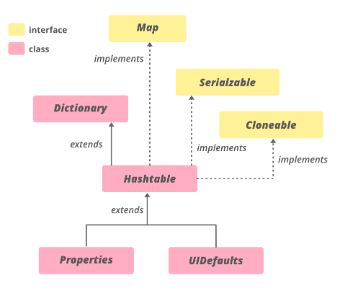GeeksforGeeks App
Open AppBrowser
Continue

# Java Program to Read Elements using Enumeration in Hashtable

The enumeration in java is one of the predefined interfaces, whose object is used for retrieving the data from collections framework variable(like Stack, Vector, HashTable, etc.) in a forward direction only and not in the backward direction.

HashTable is a class The hash table class implements a Map, which maps keys to values. It stores the key/value pair in the hash table. In this data structure we specify an object that is used as a key, and the value we want to associate with that key. The key is then hashed, and the resulting hash code is used as the index at which the value is stored within the table. HashMap doesn’t provide any Enumeration, while HashTable provides not fail-fast Enumeration. The hierarchy of the hash table is as follows:Syntax:

`public class Hashtable<K,V> extends Dictionary<K,V> implements Map<K,V>, Cloneable, Serializable`

Parameters:

• K: Keys inserted.
• V: Values assigned to the keys.

In order to create HashTable, import hash Table from java.util.Hashtable where, K, V are of data types like integer, string, float, etc.

Syntax: Creating hash Table

`Hashtable<K, V> ht = new Hashtable<K, V>();`

Implementation: College Student Information

Example

## Java

 `// Java Program to read elements``// using enumeration in hashtable` `// Importing enumeration class``import` `java.util.Enumeration;``// Importing hash table``import` `java.util.Hashtable;` `// Class``public` `class` `GFG {` `    ``// Main driver method``    ``public` `static` `void` `main(String a[])``    ``{` `        ``// Creating hash table``        ``Hashtable hm``            ``= ``new` `Hashtable();` `        ``// Add key-value pair to Hashtable``        ``// Custom inputs``        ``hm.put(``"Name"``, ``"Bahubali"``);``        ``hm.put(``"College"``, ``"Amarnath"``);``        ``hm.put(``"Department"``, ``"Vedics"``);` `        ``// enum``        ``Enumeration keys = hm.keys();` `        ``// Condition check whether element(K,V) is present``        ``// using hasMoreElements()``        ``while` `(keys.hasMoreElements()) {``            ``String key = keys.nextElement();` `            ``// Print corresponding key-value pair``            ``System.out.println(``"Value of "` `+ key``                               ``+ ``" is: "` `+ hm.get(key));``        ``}` `        ``System.out.println();` `        ``// Creating a new Hashtable``        ``Hashtable hm1``            ``= ``new` `Hashtable();` `        ``// Adding key-value pair to Hashtable``        ``// Custom inputs``        ``hm1.put(``"Name"``, ``"Ravaan"``);``        ``hm1.put(``"College"``, ``"SriLanka"``);``        ``hm1.put(``"Department"``, ``"CS"``);` `        ``// Enum``        ``Enumeration keys1 = hm1.keys();` `        ``// Condition check whether element(K,V) is present``        ``// using hasMoreElements()``        ``while` `(keys1.hasMoreElements()) {``            ``String key = keys1.nextElement();` `            ``// Print corresponding key-value pair``            ``System.out.println(``"Value of "` `+ key``                               ``+ ``" is: "` `+ hm1.get(key));``        ``}` `        ``System.out.println();` `        ``// Creating a new Hashtable``        ``Hashtable hm2``            ``= ``new` `Hashtable();` `        ``// Adding key-value pair to Hashtable``        ``// Custom inputs``        ``hm2.put(``"Name"``, ``"Kattappa"``);``        ``hm2.put(``"College"``, ``"Beardo"``);``        ``hm2.put(``"Department"``, ``"War"``);` `        ``/// enum``        ``Enumeration keys2 = hm2.keys();` `        ``// Condition check whether element(K,V) is present``        ``// using hasMoreElements()``        ``while` `(keys2.hasMoreElements()) {``            ``String key = keys2.nextElement();` `            ``// Print corresponding key-value pair``            ``System.out.println(``"Value of "` `+ key``                               ``+ ``" is: "` `+ hm2.get(key));``        ``}``    ``}``}`

Output

```Value of Name is: Bahubali
Value of College is: Amarnath
Value of Department is: Vedics

Value of Name is: Ravaan
Value of College is: SriLanka
Value of Department is: CS

Value of Name is: Kattappa
Value of College is: Beardo
Value of Department is: War```

My Personal Notes arrow_drop_up